Courses

# Test: Design For Static And Dynamic Load (Level - 2)

## 20 Questions MCQ Test Machine Design | Test: Design For Static And Dynamic Load (Level - 2)

Description
This mock test of Test: Design For Static And Dynamic Load (Level - 2) for Mechanical Engineering helps you for every Mechanical Engineering entrance exam. This contains 20 Multiple Choice Questions for Mechanical Engineering Test: Design For Static And Dynamic Load (Level - 2) (mcq) to study with solutions a complete question bank. The solved questions answers in this Test: Design For Static And Dynamic Load (Level - 2) quiz give you a good mix of easy questions and tough questions. Mechanical Engineering students definitely take this Test: Design For Static And Dynamic Load (Level - 2) exercise for a better result in the exam. You can find other Test: Design For Static And Dynamic Load (Level - 2) extra questions, long questions & short questions for Mechanical Engineering on EduRev as well by searching above.
QUESTION: 1

Solution:
QUESTION: 2

Solution:
QUESTION: 3

### A plate shown in figure subjected to force 60 kN . The stress concentration factor is 2.3 and maximum stress induced is 150 MPa, width of plate required is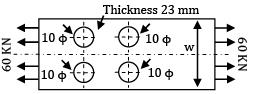Solution: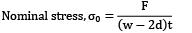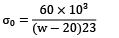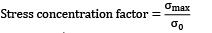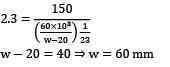w - 20 = 40 ⇒w = 60 mm

QUESTION: 4

Endurance strength in reversals load in steel is found at

Solution:
QUESTION: 5

Fatigue strength of a machine element may be improved by

Solution:
*Answer can only contain numeric values
QUESTION: 6

For a beam stress concentration factor for static load is 1.35 and stress concentration factor for fatigue load is 1.3. The notch sensitivity for specimen is __________.

Solution:

Kt = 1.35, kf = 1.3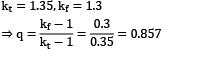QUESTION: 7

The design stress for a component subjected to a completely reversible load, is found by applying the factor of safety to

Solution:
QUESTION: 8

Auto fretting is a

Solution:
QUESTION: 9

Maximum stress induced at the edge of an elliptical hole of major axis 4 mm and minor axis 1.5 mm in a flat plate, as shown in figure, is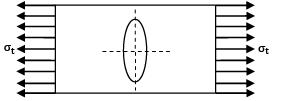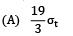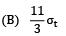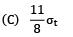Solution: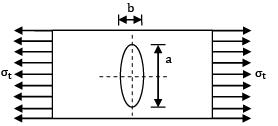a = major axis

b = minor axis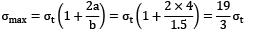QUESTION: 10

Maximum stress in the part shown in figure is induced at the section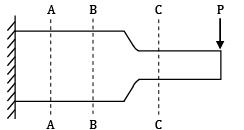Solution:
QUESTION: 11

In designing a shaft for variable loads, the S.N. diagram can be drawn by

Solution: For variable loads designing is based upon S-N curve.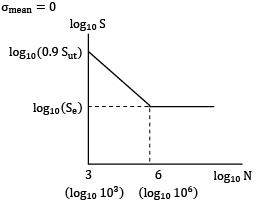*Answer can only contain numeric values
QUESTION: 12

A plate having width 120 mm is subjected to a tensile load that has a maximum value of 250 kN and a minimum value of 100 kN. The properties of the plate material are as follows.

Endurance limit stress = 225 MPa

Yield point st!ess = 300 MPa

Factor of safety (N) =.1.5

The thickness of the plate as per Soderberg equation is __________ mm

Solution:

Width (b) = 120 mm

Wmax = 250 KN, Wmin = 100 KN

Se = 225 MPa, syt = 300 MPa

N = 1.5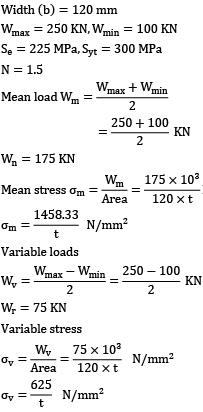Wn= 175 KNVariable Loads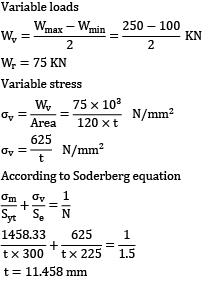Wr = 75 KN

Variable stressAccording to Soderberg equation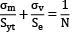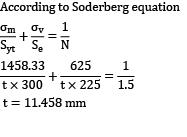t = 11.458 mm

QUESTION: 13

A loaded semi-infinite flat plate is having an elliptical hole in the middle as shown in the figure. The stress concentration factor due to the elliptical hole is?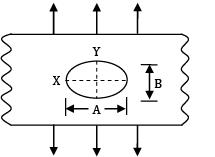Solution: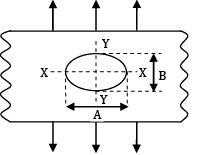Stress concentration factor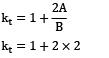Kt = 1 + 2 x 2

Kt = 5

QUESTION: 14

A thin spherical pressure vessel with 500 mm inner diameter is welded from steel plates having yield strength, σy = 200 MPa and endurance limit,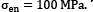a. The vessel is subjected to internal pressure which varies from 2 MPa to 6 MPa. Using Soderberg’s equation for fatigue failure and considering a factor of safety of 3, the thickness of the vessel is

Solution:

Thin spherical pressure vessel

Syt = 200 MPa

Se = 100 MPa, inner diameter d = 500 mm

Internal pressure = 2MPa

Factor of safety (N) = 3

Thickness of the vessel (t) =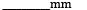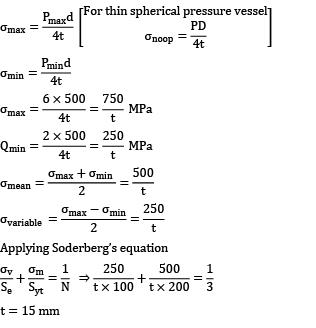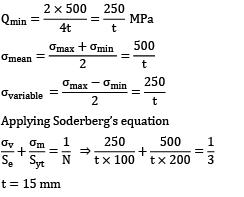Applying Soderberg’s equation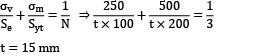t = 15 mm

QUESTION: 15

Assertion (A): Soderberg relation is used for design against fatigue.

Reason (R): Soderberg relation is based on yield strength of the material whereas all other failure relations for dynamic loading are based on ultimate strength of the material.

Solution:

Soderberg’s equation: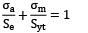For fatigue load we use Soderberg, Gerber, Goodman criteria for the design.

⇒When there is a combination of variable stress with the mean stress, then we use the above three design parameters.

⇒So, both Assertion and Reason are correct but Reason is not the correct explanation of the Assertion.

QUESTION: 16

A steel component has a theoretical stress concentration factor of 2.1 and a notch sensitivity of 0.5. Its effect on the endurance strength is

Solution:

Theoretical stress concentration factor

kt = 2.1

Notch Sensitivity (q) = 0.5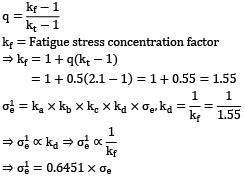kf = Fatigue stress concentration factor

⇒kf = 1 + q(kt - 1)

= 1 + 0.5(2.1 - 1) = 1 + 0.55 = 1.55Endurance strength is reduced by 35.5%

QUESTION: 17

Consider the following statements

1. Endurance strength of a component is not affected by its surface finish and notch sensitivity of the material.

2. For ferrous materials like steel, S-N curve becomes asymptotic at cycles.

Which of the statements given above is/are correct?

Solution:

Statement 1 is wrong, as the endurance strength is affected by its surface finish and notch sensitivity.

⇒If surface finish is poor, then endurance strength will decrease.

⇒If notch sensitivity(q) increases, then it will increase the fatigue stress concentration factor(kf) which will decrease the endurance strength.

QUESTION: 18

The design calculations for members subject to fluctuating loads with the same factor of safety, yield the most conservative estimates when using

Solution:
QUESTION: 19

In the figure shown, if the line AB represents Goodman criterion of failure, then Soderberg criterion could be represented by line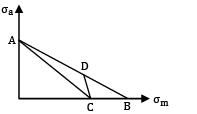Solution:
QUESTION: 20

Which of the following threaded joint will give the minimum stress concentration result?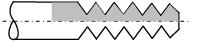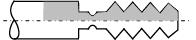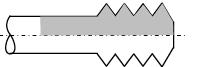Solution: Option (D) will give better result, in this, shank diameter is reduced and made equal to the core diameter of the thread. The force flow line is almost straight and there is no stress concentration.